## Leaf Area Index Using Units in Calculations

Explore using units in calculations with the Leaf Area Index (LAI). LAI is a ratio that describes the number of square meters of leaves per square meter of available land surface. Because of the units in the ratio, it is dimensionless. Values range from 0 to 7 square meters of leaf area per square meter of land surface. Values greater than one mean that the location is covered with several layers of leaves, as in a forest canopy. But how can there be more square meters of leaf than land surface in an area? Because the vegetation grows in layers above the ground. For instance, an LAI value of 5 means that there are five layers of leaves in that area. The LAI is directly related to the health and primary productivity of plants, as plants are more productive with more leaves. A value of less than 1 means that if you took all the leaves in an area and laid them flat on the ground, there wouldn't be enough to cover the land surface.

### Mini Lesson

Math Background

You can do math with units just like you do it with numbers. For example, any number divided by itself is equal to 1. The same is true of units.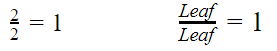Fractions made of two different units with equal magnitudes can be made into fractions equal to 1 also. For example, 1 meter = 100 cm. Since they are equal, the following fractions are equal to 1. These are called unit multipliers.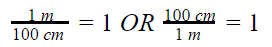When multiplying with two factors, a number and one, the answer is the factor other than one. In other words, when you multiply a number by 1, the answer is the original number. This works for fractions equal to 1.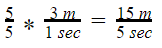Knowing this, the units can be canceled when doing calculations, just like numbers. This is shown below using several steps but can be done mentally in fewer steps.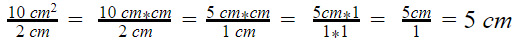This can be used with unit multipliers to convert units. The answer will have different units but it is still the same actual amount. For example, 3 m per second is equal to how many centimeters per second?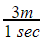needs to be converted to centimeters per second. Use the unit multiplier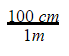and cancel units.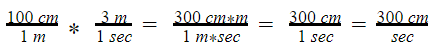This is how you determine the units for the answer to a problem. Sometimes, all the units will cancel out giving a number with no units. This often happens with ratios. LAI, or Leaf Area Index, is a ratio that demonstrates this.

Why is Leaf Area important?

Knowing the amount of leaves present at a location on Earth relative to the total land area at that location. Understanding the total leaf area in a plant canopy helps scientists infer:

• The amount of water stored and released by an ecosystem
• The value of leaf litter it generated
• The degree of photosynthesis occurring  at any given place and time

Such information also helps scientists understand the flow of energy among the various layers of vegetation, the atmosphere, and the ground, which in turn affects climate.

What is Leaf Area Index?

The Moderate Resolution Imaging Spectroradiometer (MODIS) aboard NASA's Terra and Aqua satellites collects global Leaf Area data on a daily basis as Leaf Area Index (LAI). The LAI is a ratio that describes the number of square meters of leaves per square meter of available land surface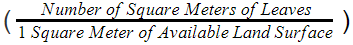. Because of the units in the ratio, it is dimensionless. Values range from 0 to 7 square meters of leaf area per square meter of land surface. Values greater than one mean that the location is covered with several layers of leaves, as in a forest canopy. But how can there be more square meters of leaf than land surface in an area? Because the vegetation grows in layers above the ground. For instance, an LAI value of 5 means that there are five layers of leaves in that area. The LAI is directly related to the health and primary productivity of plants, as plants are more productive with more leaves. A value of less than 1 means that if you took all the leaves in an area and laid them flat on the ground, there wouldn't be enough to cover the land surface.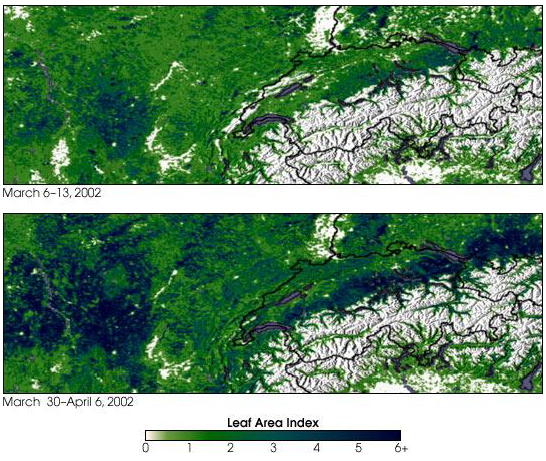LAI Example for March and April 2002 - NASA Earth Observatory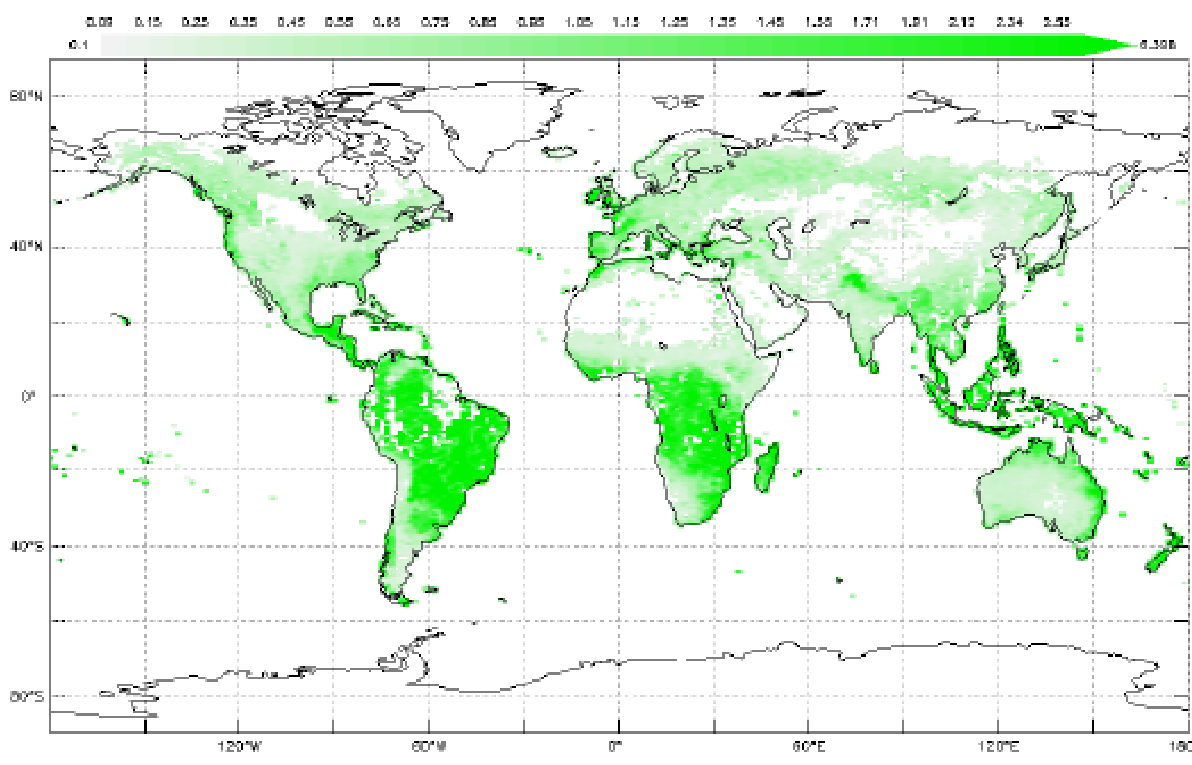LAI March 2018 - My NASA Data Earth System Data Explorer

How is Leaf Area Index Calculated?

The calculation is square meters of leaves divided by the square meters of available land surface. Here is an example.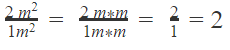This means there are two layers of leaves per square meter in the area measured. The units cancel out, so LAI is dimensionless.

Mini Lesson

1. Calculate leaf area index for the following examples. Use the units in the calculations.
•         1 m2 of leaves for 1 m2
•         3.2 m2 of leaves for 2 m2
•         100 m2 of leaves for 250 m2
2. Look at the monthly leaf index image for March 2018 and identify some areas where the LAI is at least 2. Where are they located?
3. Identify some areas where the LAI is less than 1.  Where are they located?
4. What do you predict would happen to LAI in an area if there were deforestation?

Sources/URL

Seeing Leaves in a New Light